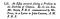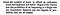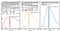# Science Behind Industry 4.0: The Origins of Bayesian Statistics

Bayesian methods have become widely used in machine learning and pattern recognition. Talking simplistically, traditional — or ‘frequentist’, statistics see probability as the limit of relative frequencies of events as the number of trials increases, assuming a fixed set of distribution parameters. Bayesian statistics, on the other hand, is more concerned with adapting a model’s parameters to observed outcomes and updating the model as more data becomes available. So, while the former approach is well suited for testing of a priori formulated hypotheses, the latter approach, with its evolving models fits, well in the kind of problems we try to solve by means of machine learning. Learning in this sense is to adapt a model to what is known and improve it by means of what becomes known over time.

As a lot has been written about all items mentioned here — the foundations and application of Bayesian methods, the fundamental debate between Bayesian and frequentist approaches, the aim of this text is try to see if what we today conceptualize as a ‘Bayesian approach’ is traceable to the original Bayesian paper (and just to be clear, a lot has been written about that too) and if thus Bayes could be seen as one of the first contributors to today’s machine learning.Figure 1: The title of the paper from the 1763 issue of the Philosophical transactions (p. 370). The F.R.S. stands for Fellow of the Royal Society which Bayes had been a member of.

The original Bayes paper “An Essay towards solving a Problem in the Doctrine of Chances”, was published posthumously in 1763 in, 2 years after Bayes’ death. It was submitted to the Royal society by Richard Price, a friend of Bayes taking care of Bayes’ legacy. Rather than just submitting the yet unpublished manuscript, Price wrote a detailed motivation of the importance of the work, emphasizing the problem Bayes addresses in his text. Moreover, Price, who himself also was a mathematician, invested considerable effort in the mathematical aspects of the paper.

Bayes open his paper with the following very clear and explicit problem description:Figure 2: Bayes’ problem description (p. 376), at the same time the first paragraph of his text.

Noting that chance here is used as synonymous for probability, what does Bayes mean by an ‘unknown event’? While obviously the event’s outcome has been observed a couple of times, ‘unknown’ relates to nothing being known in advance about what the expected outcome would be, especially nothing is known about what we today — in Bayesian terms — call the prior probability, in Bayes’ words “ the only thing we must rest on are the numbers of how often the event has happened, and how often it has failed”.

The other important term to understand is what is described in the ‘Required’ phrase. Slightly rephrasing it, Bayes wants to estimate the probability of the event to happen falling in an interval of two given (“ that can be named ‘) probabilities. If we let the interval get infinitesimally small, we would todays say that Bayes wants to know the value of the probability density distribution (PDF) of the chance of the event to happen, given an observation of a number of happened and failed events. At the time of Bayes, the term PDF was not used, instead this kind of problem was referred to as an ‘inverse probability’ problem, a term Bayes himself does not use but Richard Price refers to in his introduction.

To find the answer to this problem Bayes starts out with some statistical groundwork, defining among others, the concept of contrary (mutually exclusive) and independent events, probability (“ the correct expectation “), followed by 7 propositions, amongst which we can find a number of propositions on conditional probabilities (which could be used to derive Bayes theorem) and the probabilities for Binomial experiments (proposition 7).

The actual problem is then treated in Section II (p. 385 ff.) using a thought experiment to derive the solution. The ‘experiment’ envisions a table, onto which balls fall with a uniform distribution over the area ( “there shall be the same probability that it rests upon any one equal part of the plane as another”).Figure 3: Bayes sketch of the thought experiment (p 385). In section II, the paper contains a long reasoning about throwing a ball and relating areas where the ball may land. By this “one may give a guess whereabout it’s probability is, and by the usual methods computing the magnitudes of the areas there mentioned, see the chance that the guess is right”. Thus, the main point is not to calculate a probability, but for a “guessed” probability to estimate the chance (probability) the guessed value is correct. The guess here would be based on the observed outcomes of a number of trials.

Based on this setup Bayes arrives at a method to calculate the probability of the event’s probability falling into a specific interval, given nothing else than the record of the event happening and failing a number of times by relating areas of the table from Figure 3. The proof and derivation are cumbersome to read, partly because of somewhat different mathematical notations and concepts (e.g., ‘fluxion’ for derivative). As Richard Price added another 18 pages with explanations and examples, we can assume, that even at the time of publication Bayes text was a hard read. The result of this derivation is the “Rule 1” (p. 411ff.) which we today formulate using the Beta distribution. The figure below shows the application of “Rule 1” in Price’s examples as well as the recalculation of the results using the Beta distribution.Figure 4: The examples by Richard Price on the application of “Rule 1” or inverse probability (p. 411 ff.) and the illustration using the PDF of the Beta distribution. With an increasing number of observations from left to right, the probability of success being in a specific interval increases, meaning that we can more accurately model the outcomes of the event.

With this result, it is now possible to build a model of the probability distribution of a repeated event based only on recorded outcomes and refine the model when more data becomes available, this is what Price shows when increasing the number of observations from 11 to 44 in his examples. Given that at Bayes time the Bible of statistics was De Moivre’s “a doctrine of chances”, a book consisting of the exposition and solution of 49 statistical problems, all of the kind that they can be solved by a priori by combinatorial analysis, Bayes approach is a really radically different way of reasoning about chances. While De Moivre’s approach requires the complete knowledge of all possible outcomes in advance (which is the hard problem he solves), Bayes case is the completely opposite, namely that absolutely nothing is know in advance on the possible outcomes, rather it is his aim to determine this by resorting to empirical data, hence also the term “inverse probability” problem (backwards from observations to parameters, or from effects to causes).

So, if we look back at the initial question, namely what is special about Bayesian statistics, we can conclude that Bayes and De Moivre want to solve completely different problems, only related by them both dealing with statistical uncertainty. Bayes approach — to use empirical data to estimate statistical models and to refine them once more data becomes available — is thus clearly traceable to his original paper. In that sense, Bayes (and Richard Price) can be seen pioneering a method crucial for many powerful and popular machine learning methods.

Bayes, Mr; Price, Mr (1763), “An Essay towards Solving a Problem in the Doctrine of Chances. Philosophical Transactions of the Royal Society of London. Vol. 53. Link.

Abraham De Moivre (1781), The Doctrine of Chances. Link.

Bishop, Christopher M. (Springer 2006), Pattern Recognition and Machine Learning. Link.

Fienberg, S. Bayesian Analysis (2006), When Did Bayesian Inference Become Bayesian? Link.

Stigler, Stephen R. Statistical Science (2018), Richard Price, the Bayesian. Link.

Repo with pdf of original paper and R-code for example plot. Link.